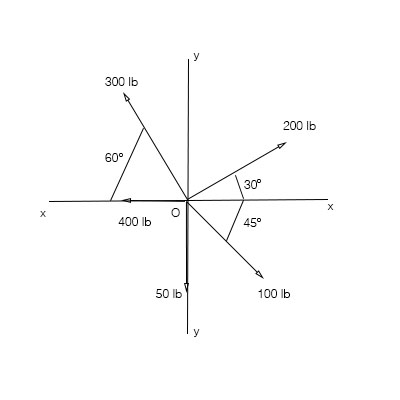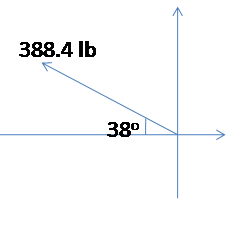# Determine the resultant of the coplanar concurrent force system shown. Compute the magnitude,...

## Question:

Determine the resultant of the coplanar concurrent force system shown. Compute the magnitude, sense, and angle of inclination with the {eq}x {/eq} axis. Use the method of components.## Force system:

When several force vectors are present in a system, then it is called a force system.

If the force vectors meet at a point then they are called concurrent.

The vectors which lie in a single plane are called coplanar vectors.

The force vector can be written in component form along the two mutually perpendicular axes.

The following vectors are given with their respective vectors,

200 lb at 30 degrees in the anticlockwise direction. with x-axis,

100 lb at 45 degrees in clockwise with the positive x-axis,

50 lb along the negative y-axis,

400 lb along the negative x-axis,

300 lb at 60 degrees in the clockwise direction with the negative x-axis.

Now the given vectors can be written in the vector form as,

{eq}200\ cos\ 30^o \hat i+ 200\ sin\ 30^o\hat j \\ 100\ cos\ 45^o\hat i -100\ sin\ 45^o\hat j\\ - 50\hat j\\ - 400\hat i\\ -300\ cos\ 60^o\hat i + 300\ sin\ 60^o\hat j \\ {/eq}

The resultant of all vectors is

{eq}\vec R = 200\ cos\ 30^o \hat i+ 200\ sin\ 30^o\hat j + 100\ cos\ 45^o\hat i -100\ sin\ 45^o\hat j - 50\hat j - 400\hat i -300\ cos\ 60^o\hat i + 300\ sin\ 60^o\hat j \\ \vec R = 173.2\hat i + 100\hat j + 70.7\hat i - 70.7\hat j - 50\hat j - 400\hat i -150\hat i + 259.8\hat j \\ \vec R = -306.1\hat i +239.1\hat j\\ {/eq}

The magnitude of the resultant is, {eq}R = \sqrt{306.1^2+ 239.1^2} = 388.4\ lb {/eq}The angle is, {eq}tan^{-1}\dfrac{239.1}{-306.1} = -37.99^o {/eq} with the negative x axis.

or {eq}142^o {/eq} with the positive x axis in the counterclockwise drection